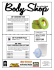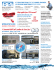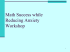# Activity 5.6 Physical Property Analysis

## Transcription

Activity 5.6 Physical Property Analysis
```Andrew Smith
Activity 5.6 Physical Property Analysis
Introduction
What do you need to know about a product before it is built? Would you need to
know its volume, surface area, or weight? Would the product weigh less if it were
made of aluminum or mild steel? What about copper, brass, or cast iron? How could
this information impact the product design?
How can you find the properties of a product before it is built? You can calculate
them mathematically, providing you have the material specifications, but it will take
time. In today’s busy, fast-paced world, engineers use solid modeling software
programs to speed up the calculating process. However, the user of the software
must understand what the software is doing in order to estimate the answers and to
be able to recognize a possible error.
Equipment




Engineering notebook
Pencil
Computer with 3D CAD solid modeling software
Calculator
Procedure
In this activity you will calculate the volume of a part and the surface area; you will
look up the density of the material and then calculate the mass. Next, you will check
your work using a 3D solid modeling software program. After you have learned how
to calculate the physical properties of the example parts provided, you will then do
an analysis on a puzzle cube piece and parts of your Automoblox vehicle or other
consumer product.
Introduction to Engineering Design Activity 5.6 Physical Property Analysis – Page 1
Aluminum Object Example 1
The aluminum example is provided as an isometric drawing that will need to be
created as a solid model. The grid spacing for the object shown is 0.25 inch. Using
the grid, determine the measurements for each facet of the part. Recreate the
isometric sketch and record each facet’s dimensions in your engineering notebook.
Then calculate the volume and surface area of the object. Show your work in your
engineering notebook. Next, create a model of the object with the required geometry
using the 3D solid modeling software. You will assign aluminum as the object’s
material and perform a physical property analysis to determine the answers to the
questions. Print a copy of the CAD Physical property values and insert into your
engineering notebook. When you have completed the physical property analysis,
You may need to review your notes and the Physical Properties PowerPoint to recall
how to generate the physical properties of a part.
Figure 1: Isometric drawing of Aluminum Object One with 0.25” grid
Introduction to Engineering Design Activity 5.6 Physical Property Analysis – Page 2
Andrew Smith
Questions for Aluminum Object One
Directions: Complete the calculation by hand. Show all work below and select the
modeling software. If your answers differ, explain why you think they are different
where appropriate.
1. What is the volume of the part?
A.
B.
C.
D.
1.875 in.³
1.125 in.³
1.375 in.³ THIS ONE
2.125 in.³
SHOW WORK:
I cannot if I want to save as an IPT! Answers would not appear on camera, either.
2. What is the density of aluminum in grams per cubic centimeter? (This may
require research. Be sure to document your source.)
All of these answers were helped with aqua-calc.com
2.69 (up) g/cm3
3. What is the density of aluminum in pounds per cubic inch? Show your work
including all conversion factors.
.0975 lb/in3
4. Find the mass of the object (in pounds-mass) if it is made of aluminum.
A.
0.125 lbm
B.
0.135 lbm THIS ONE
C.
0.257 lbm
D.
0.312 lbm
SHOW WORK:
#1
5. What is the surface area of the part?
A.
B.
C.
D.
7.250 in.²
8.250 in.²
9.250 in.²
10.250 in.² This one
Introduction to Engineering Design Activity 5.6 Physical Property Analysis – Page 3
SHOW WORK:
#1
6. What are the physical properties presented in the CAD software? Include units.
A. Density __2.7 g/cm3_____________
B. Mass __.134 lb_________mass____
C. Surface Area _10.25 in2______________
D. Volume _____1.375 in3__________
If physical properties are different from hand calculated values, explain why you
think they differ.
Human error!
7. If one quart of cleaning solution will clean 14400 in. 2, how many quarts will be
required to clean 3000 parts? Use 3D software physical properties.
A.
B.
C.
D.
2.000
1.500
2.135 This one
2.145
SHOW WORK:
#1
Brass Object Example 2
An isometric drawing of a brass part is provided. The grid spacing for the object
shown is 0.25 inch. Using the grid, determine the measurements for each facet of
the part. Recreate the isometric sketch and record each facet’s dimensions in your
engineering notebook. Calculate the volume, mass, and surface area of the object
and record below. Next, create a model of the object with the required geometry
using the 3D solid modeling software. You will assign brass as the object’s material
and perform a physical property analysis to determine the answers to the questions.
Print a copy of the CAD physical property values and insert into your engineering
Introduction to Engineering Design Activity 5.6 Physical Property Analysis – Page 4
Andrew Smith
notebook. When you have completed the physical property analysis, answer the
questions below.
Figure 2: Isometric drawing of Brass Object One with 0.25” grid
Questions for Brass Object One
Directions: Complete the calculation by hand. Show all work below and select the
modeling software. If your answers differ, explain why you think they are different
where appropriate.
1. What is the volume of the part?
A.
B.
C.
D.
2.375 in.³
3.125 in.³
2.031 in.³ This one
4.125 in.³
SHOW WORK:
#1
8. What is the density of brass in grams per cubic inch?
Varies-is alloy.
Introduction to Engineering Design Activity 5.6 Physical Property Analysis – Page 5
9. What is the density of brass in pounds per cubic inch? Show your work including
all conversion factors.
Varies-is alloy.
10. Find the mass of the object if it is made of brass.
A.
B.
C.
D.
0.621 lbm This one
0.547 lbm
0.257 lbm
0.312 lbm
SHOW WORK:
#1
11. What is the surface area of the part?
A.
B.
C.
D.
6.000 in.²
8.000 in.²
0.250 in.²
12.000 in.² This one
SHOW WORK:
#1
12. What are the physical properties presented in the CAD software? Include units.
a.
Density _8.47 g/cm3______________
b.
Mass ____________.622 lb mass_______
c.
Surface Area ______12 in2_____________
d. Volume ___2.031 in3________________
If physical properties are different from hand-calculated values, explain why you
think they differ.
Human error OR the fact it's an alloy.
Introduction to Engineering Design Activity 5.6 Physical Property Analysis – Page 6
Andrew Smith
13. What will be the total cost to ship 100 brass parts if the shipping rate is \$4.25 per
pound? Use the physical properties from the CAD analysis. SHOW WORK.
\$14.64
Cannot show work-#1
High Density Polyethylene Example 3
You will begin by using Figure 2 isometric drawing to create a solid model. The grid
spacing for the object shown is 1 cm. Note that this part has different dimensions
(using metric units) than the similar part you created in Activity 5.5b CAD Modeling
Skills Part 2. Recreate the isometric sketch and record each facet’s dimensions in
your engineering notebook. Next, create a model of the object with the required
geometry using the 3D solid modeling software. (Hint: Use subtractive modeling by
creating a rectangular solid, and then use the Loft tool to cut the V-notch.)
Remember to use a metric part file template. Assign high density polyethylene as the
object’s material and perform a physical property analysis. Insert a copy of the CAD
When you have completed the physical property analysis, answer the questions
below.
Figure 3: Isometric drawing of High Density Polyethylene Object using a 1 cm grid
1. What are the physical properties presented in the CAD software? Include units.
A. Density ______________.952 g/cm3_____
B. Mass _______________.136 kg mass____
C. Surface Area _____________19419.4 (repeat)______
D. Volume _________143333.3 (repeat) ________
Introduction to Engineering Design Activity 5.6 Physical Property Analysis – Page 7
Introduction to Engineering Design Activity 5.6 Physical Property Analysis – Page 8
Andrew Smith
14. What is the surface area in square centimeters? SHOW WORK.
194.19 cm2
15. What is the volume in cubic centimeters? SHOW WORK.
143.3 (repeat)
16. What is the weight of the high density polyethylene part in pounds? Show your
work and include all conversion factors.
.3 lbs
17. How many parts can be made from 152 pounds of high density polyethylene?
SHOW WORK.
506, actual number is 506.6 (repeat)
Conclusion
1. What do you need to know in order to perform a physical property analysis?
You need to know the dimensions and material of an object
2. Why is it important to understand the mathematics used in physical property
analysis?
It can affect shipment and production and use of an object
3. Why is it important to perform a physical property analysis prior to producing a
part?
It is important to realize if your equipment can even handle such high properties.
Introduction to Engineering Design Activity 5.6 Physical Property Analysis – Page 9
4. The clear enclosure piece and the wheels of the Automoblox are manufactured
from polycarbonate. Do you think that the connector pieces are also
I would imagine so, if only because it saves money. A
completely adhesive material would only harm it eventually,
so just having some more polycarbonate wouldn't hurt.
Introduction to Engineering Design Activity 5.6 Physical Property Analysis – Page 10
```

### ACANTHUS TOWEL RING | 3430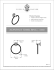### LynZoil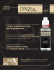### poster - Reed College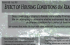### Sales sheet - World Music Network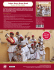### IsoMetrix Information Sheet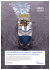### Cox London for Rose Tarlow Melrose House #WL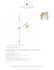### Alumex - xintex group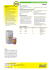### see Complete Details - Auto Refinish Solutions